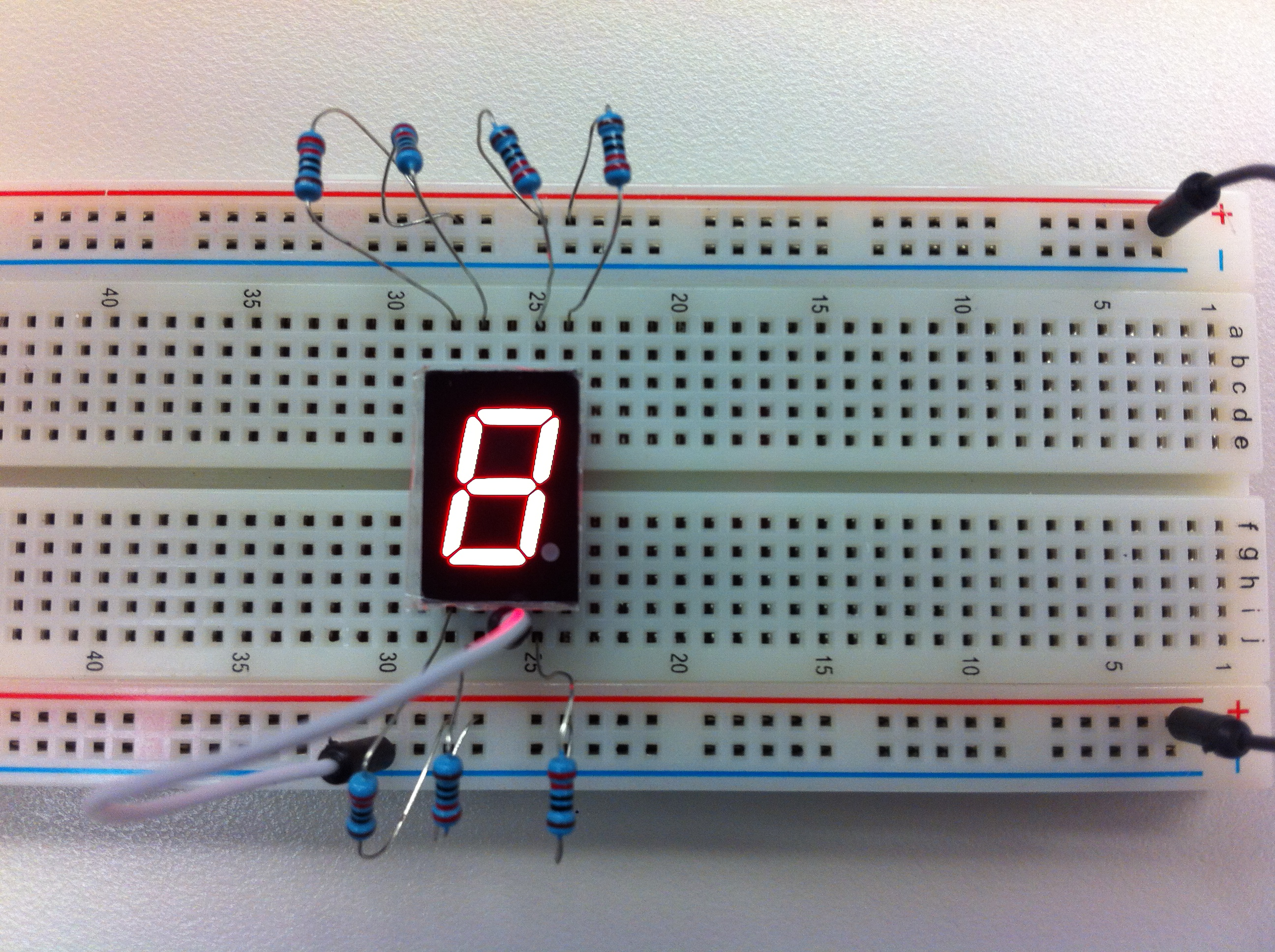# Arduino and the 7 Segment LED

In this assignment you are going to get a 7 segment LED to count from 1 to 9.
You might need to go back and review how to hook up the 7 segment LED.Some of the code that you might need is here:

This first bit of code sets up the 7 LEDs and their ports in the arduino...

int led1 = 13;
int led2 = 12;
int led3 = 11;
int led4 = 10;
int led5 = 9;
int led6 = 8;
int led7 = 7;

void setup()
{
pinMode(led1, OUTPUT);
pinMode(led2, OUTPUT);
pinMode(led3, OUTPUT);
pinMode(led4, OUTPUT);
pinMode(led5, OUTPUT);
pinMode(led6, OUTPUT);
pinMode(led7, OUTPUT);
}

This code will make LEDs 1 and 5 light up for a second and then turn off (this will generate the number 1)...

void loop()
{
digitalWrite(led1, HIGH);
digitalWrite(led5, HIGH);
delay(1000);
digitalWrite(led1, LOW);
digitalWrite(led5, LOW);
}